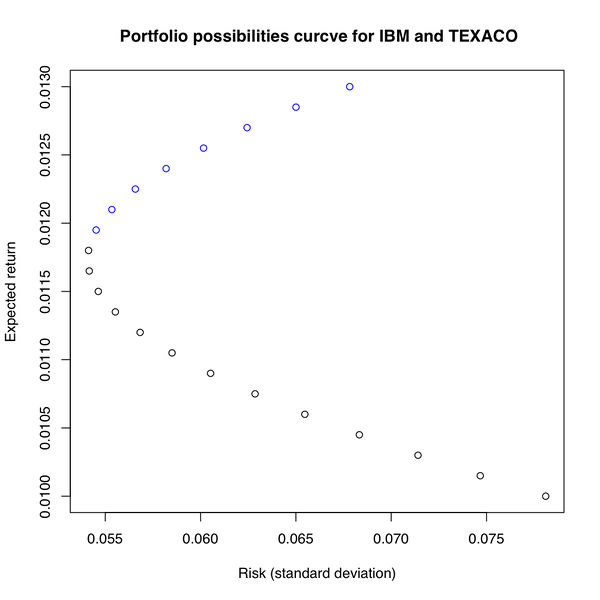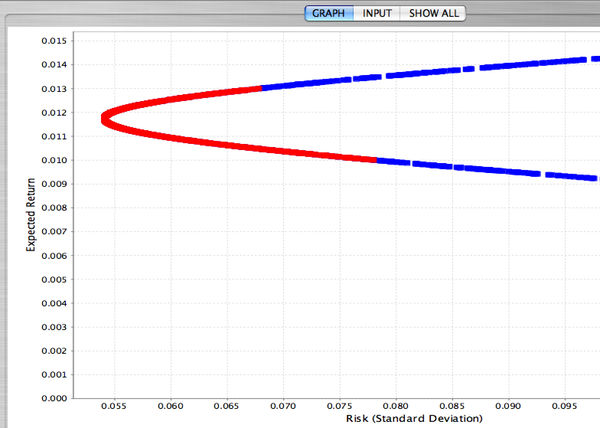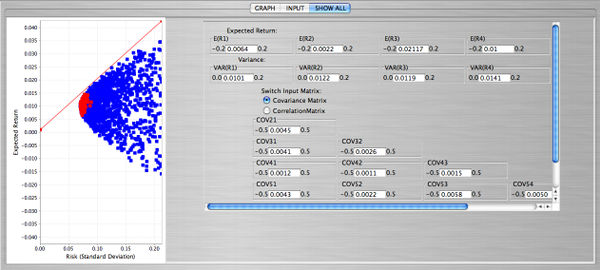# Difference between revisions of "SOCR EduMaterials Activities ApplicationsActivities Portfolio"

## SOCR Applications Activities - Portfolio Theory

### Description

You can access the portfolio applet at the SOCR Applications Site, select Financial Applications --> Portfolio.

• An investor has a certain amount of dollars to invest into two stocks ($$IBM$$ and $$TEXACO$$). A portion of the available funds will be invested into IBM (denote this portion of the funds with $$x_A$$) and the remaining funds into TEXACO (denote it with $$x_B$$) - so $$x_A+x_B=1$$. The resulting portfolio will be $$x_A R_A+x_B R_B$$, where $$R_A$$ is the monthly return of $$IBM$$ and $$R_B$$ is the monthly return of $$TEXACO$$. The goal here is to find the most efficient portfolios given a certain amount of risk.
Using market data from January 1980 until February 2001 we compute that $$E(R_A)=0.010$$, $$E(R_B)=0.013$$, $$Var(R_A)=0.0061$$, $$Var(R_B)=0.0046$$, and $$Cov(R_A,R_B)=0.00062$$. We first want to minimize the variance of the portfolio. This will be:

$\mbox{Minimize} \ \ \mbox{Var}(x_A R_A+x_BR_B)$

$\mbox{subject to} \ \ x_A+x_B=1$

Or

$\mbox{Minimize} \ \ x_A^2 Var(R_A)+x_B^2 Var(R_B) + 2x_Ax_BCov(R_A,R_B)$ $\mbox{subject to} \ \ x_A+x_B=1$

• Therefore our goal is to find $$x_A$$ and $$x_B$$, the percentage of the available funds that will be invested in each stock. Substituting $$x_B=1-x_A$$ into the equation of the variance we get

$x_A^2 Var(R_A)+(1-x_A)^2 Var(R_B) + 2x_A(1-x_A)Cov(R_A,R_B).$

• To minimize the above expression we take the derivative with respect to $$x_A$$, set it equal to zero and solve for $$x_A$$. The result is:

$x_A=\frac{Var(R_B) - Cov(R_A,R_B)}{Var(R_A)+Var(R_B)-2Cov(R_A,R_B)}$,

and therefore

$x_B=\frac{Var(R_A) - Cov(R_A,R_B)}{Var(R_A)+Var(R_B)-2Cov(R_A,R_B)}$.

The values of $$x_A$$ and $$x_B$$ are:

$x_A=\frac{0.0046-0.0062}{0.0061+0.0046-2(0.00062)} \Rightarrow x_A=0.42,$

and $$x_B=1-x_A=1-0.42 \Rightarrow x_B=0.58$$. Therefore, if the investor invests $$42 \%$$ of the available funds into $$IBM$$ and the remaining $$58 \%$$ into $$TEXACO$$ the variance of the portfolio will be minimum and equal to:

$Var(0.42R_A+0.58R_B)=0.42^2(0.0061)+0.58^2(0.0046)+2(0.42)(0.58)(0.00062)=0.002926.$

The corresponding expected return of this portfolio will be:

$E(0.42R_A+0.58R_B)=0.42(0.010)+0.58(0.013)=0.01174.$

• We can try many other combinations of $$x_A$$ and $$x_B$$ (but always $$x_A+x_B=1$$) and compute the risk and return for each resulting portfolio. This is shown in the table and the graph below.
$$x_A$$ $$x_B$$ Risk ($$\sigma^2$$) Return Risk ($$\sigma$$)
1.00 0.00 0.006100 0.01000 0.078102
0.95 0.05 0.005576 0.01015 0.074670
0.90 0.10 0.005099 0.01030 0.071404
0.85 0.15 0.004669 0.01045 0.068329
0.80 0.20 0.004286 0.01060 0.065471
0.75 0.25 0.003951 0.01075 0.062859
0.70 0.30 0.003663 0.01090 0.060526
0.65 0.35 0.003423 0.01105 0.058505
0.60 0.40 0.003230 0.01120 0.056830
0.55 0.45 0.003084 0.01135 0.055531
0.50 0.50 0.002985 0.01150 0.054635
0.42 0.58 0.002926 0.01174 0.054088
0.40 0.60 0.002930 0.01180 0.054126
0.35 0.65 0.002973 0.01195 0.054524
0.30 0.70 0.003063 0.01210 0.055348
0.25 0.75 0.003201 0.01225 0.056580
0.20 0.80 0.003386 0.01240 0.058193
0.15 0.85 0.003619 0.01255 0.060157
0.10 0.90 0.003899 0.01270 0.062439
0.05 0.95 0.004226 0.01285 0.065005
0.00 1.00 0.004600 0.01300 0.067823• For the above calculations short selling was not allowed ($$0 \le x_A \le 1$$ and $$0 \le x_B \le 1$$, in addition to $$x_A+x_B=1$$). We note here that the efficient portfolios are located on the top part of the graph between the minimum risk portfolio point and the maximum return portfolio point, which is called the efficient frontier (the blue portion of the graph). Efficient portfolios should provide higher expected return for the same level of risk or lower risk for the same level of expected return.
• If short sales are allowed, which means that the investor can sell a stock that he or she does not own the graph has the same shape but now with more possibilities. The investor can have very large expected return but this will be associated with very large risk. The constraint here is only $$x_A+x_B=1$$, since either $$x_A$$ or $$x_B$$ can be negative. The snapshot below from the SOCR applet shows the short sales scenario" for the IBM and TEXACO stocks. The blue portion of the portfolio possibilities curve occurs when short sales are allowed, while the red portion corresponds to the case when short sales are not allowed.• When the investor faces the efficient frontier when short sales are allowed and he or she can lend or borrow at the risk-free interest rate the efficient frontier will change in the following way: Let $$x$$ be the portion of the investor's wealth invested in portfolio $$A$$ that lies on the efficient frontier, and $$1-x$$ the the portion invested in a risk-free asset. This combination is a new portfolio and has

$\bar R_p=x\bar R_A + (1-x)R_f$
where $$R_f$$ is the return of the risk-free asset. The variance of this combination is simply $\sigma_p^2=x^2 \sigma_A^2 \Rightarrow x=\frac{\sigma_p}{\sigma_A}$
From the last two equations we get $\bar R_p = R_f + \left(\frac{\bar R_A-R_f}{\sigma_A}\right)\sigma_p$

• This is an equation of a straight line. On this line we find all the possible combinations of portfolio $$A$$ and the risk-free rate. Another investor can choose to combine the risk-free rate with portfolio $$B$$ or portfolio $$C$$. Clearly, for the same level risk the combinations that lie on the $$Rf-B$$ line have higher expected return than those on the line $$Rf-A$$ (see figure below). And $$Rf-C$$ will produce combinations that have higher return than those on $$Rf-B$$ for the same level of risk, etc.
Error creating thumbnail: File missing
• The solution, therefore, is to find the point of tangency of this line to the efficient frontier. Let's call this point $$G$$. To find this point we want to maximize the slope of the line in (1) as follows:

$\mbox{max} \ \ \theta = \frac{\bar R_p - R_f}{\sigma_p}$

Subject to

$\sum_{i=1}^{n} x_i = 1$

Since,

$R_f=\left(\sum_{i=1}^n x_i\right) R_f = \sum_{i=1}^n x_iR_f$

• We can write the maximization problem as

$\mbox{max} \ \ \theta=\frac{\sum_{i=1}^n x_i (\bar R_i - R_f)} {\left(\sum_{i=1}^n x_i^2 \sigma_i^2 + \sum_{i=1}^n \sum_{j=1, j \ne i}^n x_i x_j \sigma_{ij}\right)^{\frac{1}{2}}}$

or

$\mbox{max} \ \ \theta=\left[\sum_{i=1}^n x_i (\bar R_i - R_f)\right] \left[\sum_{i=1}^n x_i^2 \sigma_i^2 + \sum_{i=1}^n \sum_{j=1, j \ne i}^n x_i x_j \sigma_{ij}\right]^{-\frac{1}{2}}$

• Take now the partial derivative with respect to each $$x_i, i=1, \cdots, n$$, set them equal to zero and solve. Let's find the partial derivative w.r.t. $$x_k$$:

$\frac{\partial \theta}{\partial x_k} = (\bar R_k - R_f)\left[\sum_{i=1}^n x_i^2 \sigma_i^2 + \sum_{i=1}^n \sum_{j=1, j \ne i}^n x_i x_j \sigma_{ij}\right]^{-\frac{1}{2}} + \left[\sum_{i=1}^n x_i(\bar R_i - R_f)\right] \left[2x_k\sigma_k^2 + 2 \sum_{j=1, j \ne k}^n x_j \sigma_{kj}\right] \times \left[\sum_{i=1}^n x_i^2 \sigma_i^2 + \sum_{i=1}^n \sum_{j=1, j \ne i}^n x_i x_j \sigma_{ij}\right]^{-\frac{3}{2}} \times (-\frac{1}{2}) = 0$

• Multiply both sides by

$\left[\sum_{i=1}^n x_i^2 \sigma_i^2 + \sum_{i=1}^n \sum_{j=1, j \ne i}^n x_i x_j \sigma_{ij}\right]^{\frac{1}{2}} \ \ \ \mbox{to get}$ $(\bar R_k - R_f) - \frac{\sum_{i=1}^n x_i(\bar R_i - R_f)} {\sum_{i=1}^n x_i^2 \sigma_i^2 + \sum_{i=1}^n \sum_{j=1, j \ne i}^n x_i x_j \sigma_{ij}} (x_k \sigma_k^2 +\sum_{j=1, j \ne k}^n x_j \sigma_{kj}) =0$

• Now, if we let

$\lambda= \frac{\sum_{i=1}^n x_i(\bar R_i - R_f)} {\sum_{i=1}^n x_i^2 \sigma_i^2 + \sum_{i=1}^n \sum_{j=1, j \ne i}^n x_i x_j \sigma_{ij}}$

the previous expression will be

$(\bar R_k - R_f) - \lambda x_k \sigma_k^2 - \sum_{j=1, j \ne k}^n \lambda x_j \sigma_{kj} = 0$

or

$\bar R_k - R_f = \lambda x_k \sigma_k^2 + \sum_{j=1, j \ne k}^n \lambda x_j \sigma_{kj}$

• Let's define now a new variable,

$z_k = \lambda x_k$

and finally

$\bar R_k - R_f = z_k \sigma_k^2 + \sum_{j=1, j \ne k}^n z_j \sigma_{kj}$

• We have one such equation for each $$k=1, \cdots, n$$. Here they are:

$\bar R_1 - R_f = z_1 \sigma_1^2 + z_2 \sigma_{12} + z_3 \sigma_{13} + \cdots + z_n \sigma_{1n}$

$\bar R_2 - R_f = z_1 \sigma_{21} + z_2 \sigma_2^2 + z_3 \sigma_{23} + \cdots + z_n \sigma_{2n}$

$\cdots \cdots$ $\cdots \cdots$ $\cdots \cdots$

$\bar R_n - R_f = z_1 \sigma_{n1} + z_2 \sigma_{n2} + z_3 \sigma_{n3} + \cdots + z_n \sigma_n^2$

• The solution involves solving the system of these simultaneous equations, which can be written in matrix form as:

$\bar R = \Sigma Z$

where $$\Sigma$$ is the variance-covariance matrix of the returns of the $$n$$ stocks. To solve for $$Z$$:

${Z} = \Sigma^{-1} \bar R$

• Once we find the $$z_i's$$ it is easy to find the $$x_i's$$ (the fraction of funds to be invested in each security). Earlier we defined

$z_k = \lambda x_k \Rightarrow x_k = \frac{z_k}{\lambda}$

• We need to find $$\lambda$$ as follows:

$z_1 + z_2 + \cdots + z_n = \sum_{i=1}^n z_i$

$\lambda(x_1 + x_2 + \cdots + x_3) = \sum_{i=1}^n z_i$ $\Rightarrow \lambda = \sum_{i=1}^n z_i$

• Therefore,

$x_1 = \frac{z_1}{\lambda}$ $x_2 = \frac{z_2}{\lambda}$

$x_3 = \frac{z_3}{\lambda}$ $\cdots$ $\cdots$ $\cdots$ $x_n = \frac{z_n}{\lambda}$

• The snapshot form the SOCR portfolio applet shows an example with 5 stocks. Again, the red points in the applet correspond to the case when short sales are not allowed. The point of tangency can be found with a choice of the risk-free rate that can be entered in the input dialog box.